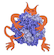IMP  2.4.0 The Integrative Modeling Platform
IMP::kernel::SingletonScore Class Reference

Abstract class for scoring object(s) of type Particle. More...

#include <IMP/kernel/SingletonScore.h>Inheritance diagram for IMP::kernel::SingletonScore:

Detailed Description

Abstract class for scoring object(s) of type Particle.

SingletonScore will evaluate the score and derivatives for passed object(s) of type Particle.

Use in conjunction with various restraints such as IMP::core::SingletonsRestraint or IMP::core::SingletonRestraint. The restraints couple the score functions with appropariate lists of object(s) of type Particle.

Implementers should check out IMP_SINGLETON_SCORE().

PredicateSingletonRestraint

Definition at line 38 of file kernel/SingletonScore.h.

Public Types

typedef kernel::ParticleArgument

typedef kernel::ParticleIndex IndexArgument

typedef SingletonModifier Modifier

typedef kernel::ParticlePassArgument

typedef kernel::ParticleIndex PassIndexArgument

Public Member Functions

SingletonScore (std::string name="SingletonScore %1%")

Restraints create_current_decomposition (kernel::Model *m, kernel::ParticleIndex vt) const

virtual double evaluate (kernel::Particle *vt, DerivativeAccumulator *da) const
Compute the score and the derivative if needed. More...

virtual double evaluate_if_good_index (kernel::Model *m, kernel::ParticleIndex vt, DerivativeAccumulator *da, double max) const
Compute the score and the derivative if needed. More...

virtual double evaluate_if_good_indexes (kernel::Model *m, const kernel::ParticleIndexes &o, DerivativeAccumulator *da, double max, unsigned int lower_bound, unsigned int upper_bound) const

virtual double evaluate_index (kernel::Model *m, kernel::ParticleIndex vt, DerivativeAccumulator *da) const
Compute the score and the derivative if needed. More...

virtual double evaluate_indexes (kernel::Model *m, const kernel::ParticleIndexes &o, DerivativeAccumulator *da, unsigned int lower_bound, unsigned int upper_bound) const
Compute the score and the derivative if needed over a set. More...Public Member Functions inherited from IMP::kernel::ParticleInputs
virtual ContainersTemp get_input_containers (Particle *p) const

virtual ParticlesTemp get_input_particles (Particle *p) const

ModelObjectsTemp get_inputs (kernel::Model *m, const ParticleIndexes &pis) constPublic Member Functions inherited from IMP::base::Object
virtual void clear_caches ()

CheckLevel get_check_level () const

LogLevel get_log_level () const

virtual VersionInfo get_version_info () const
Get information about the module and version of the object. More...

void set_check_level (CheckLevel l)

void set_log_level (LogLevel l)
Set the logging level used in this object. More...

void set_was_used (bool tf) const

void show (std::ostream &out=std::cout) const

const std::string & get_name () const

void set_name (std::string name)

virtual std::string get_type_name () const

Protected Member Functions

virtual Restraints do_create_current_decomposition (kernel::Model *m, kernel::ParticleIndex vt) constProtected Member Functions inherited from IMP::kernel::ParticleInputs
virtual ModelObjectsTemp do_get_inputs (kernel::Model *m, const ParticleIndexes &pis) constProtected Member Functions inherited from IMP::base::Object
Object (std::string name)
Construct an object with the given name. More...

virtual void do_destroy ()

Member Function Documentation

 Restraints IMP::kernel::SingletonScore::create_current_decomposition ( kernel::Model * m, kernel::ParticleIndex vt ) const

Decompose this pair score acting on the pair into a set of restraints. The scoring function and derivatives should be equal to the current score. The defualt implementation just returns this object bound to the pair.

 virtual Restraints IMP::kernel::SingletonScore::do_create_current_decomposition ( kernel::Model * m, kernel::ParticleIndex vt ) const
protectedvirtual

Overide this to return your own decomposition.

Reimplemented in IMP::container::MinimumSingletonScore.

 virtual double IMP::kernel::SingletonScore::evaluate ( kernel::Particle * vt, DerivativeAccumulator * da ) const
virtual

Compute the score and the derivative if needed.

Deprecated:
 virtual double IMP::kernel::SingletonScore::evaluate_if_good_index ( kernel::Model * m, kernel::ParticleIndex vt, DerivativeAccumulator * da, double max ) const
virtual

Compute the score and the derivative if needed.

Compute the score and the derivative if needed as in evaluate_index(). but may terminate the computation early if the score is higher than max.

Returns
the score if score<= max or some arbitrary value > max otherwise.
 virtual double IMP::kernel::SingletonScore::evaluate_if_good_indexes ( kernel::Model * m, const kernel::ParticleIndexes & o, DerivativeAccumulator * da, double max, unsigned int lower_bound, unsigned int upper_bound ) const
virtual

Compute the score and the derivative if needed as in evaluate_index(). but may terminate the computation early if the total score is higher than max.

Returns
the score if score<= max or some arbitrary value > max otherwise.

Implementations for these are provided by the IMP_SINGLETON_SCORE() macro.

 virtual double IMP::kernel::SingletonScore::evaluate_index ( kernel::Model * m, kernel::ParticleIndex vt, DerivativeAccumulator * da ) const
virtual

Compute the score and the derivative if needed.

evaluate the score and the derivative if needed over vt

Parameters
 m the model of vt vt the index in m of an object of type Particle da a derivative accumulator that reweighting computed derivatives. If nullptr, derivatives will not be computed
 virtual double IMP::kernel::SingletonScore::evaluate_indexes ( kernel::Model * m, const kernel::ParticleIndexes & o, DerivativeAccumulator * da, unsigned int lower_bound, unsigned int upper_bound ) const
virtual

Compute the score and the derivative if needed over a set.

evaluate the score and the derivative if needed over a set of objects in o

Parameters
 m the model of o o objects of type Particle, specified by index da a derivative accumulator that reweighting computed derivatives. If nullptr, derivatives will not be computed lower_bound index of first item in o to evaluate upper_bound index of last item in o to evaluate
Note
Implementations for these are provided by the IMP_SINGLETON_SCORE() macro.

The documentation for this class was generated from the following file: